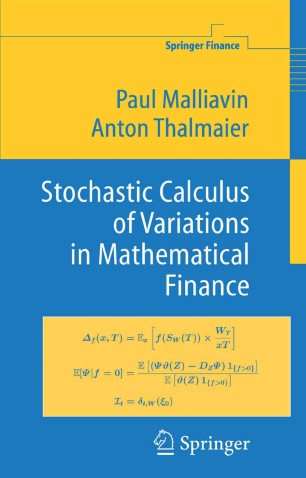# Stochastic Calculus of Variations in Mathematical Finance

• Paul Malliavin
• Anton ThalmaierBook

Part of the Springer Finance book series (FINANCE)

1. Front Matter
Pages I-XI
2. Pages 77-85
3. Pages 87-96
4. Back Matter
Pages 107-142

### Introduction

Malliavin calculus provides an infinite-dimensional differential calculus in the context of continuous paths stochastic processes. The calculus includes formulae of integration by parts and Sobolev spaces of differentiable functions defined on a probability space. This new book, demonstrating the relevance of Malliavin calculus for Mathematical Finance, starts with an exposition from scratch of this theory. Greeks (price sensitivities) are reinterpreted in terms of Malliavin calculus. Integration by parts formulae provide stable Monte Carlo schemes for numerical valuation of digital options. Finite-dimensional projections of infinite-dimensional Sobolev spaces lead to Monte Carlo computations of conditional expectations useful for computing American options. The discretization error of the Euler scheme for a stochastic differential equation is expressed as a generalized Watanabe distribution on the Wiener space. Insider information is expressed as an infinite-dimensional drift. The last chapter gives an introduction to the same objects in the context of jump processes where incomplete markets appear.

### Keywords

American option Insider information Malliavin calculus Market equilibrium Monte Carlo weight Price sensitivity Stochastic Processes Stochastic calculus Volatility measurement calculus

#### Authors and affiliations

• Paul Malliavin
• 1
• Anton Thalmaier
• 2
1. 1.Académie des SciencesInstitut de FranceFrance
2. 2.Département de MathématiquesUniversité de PoitiersFrance